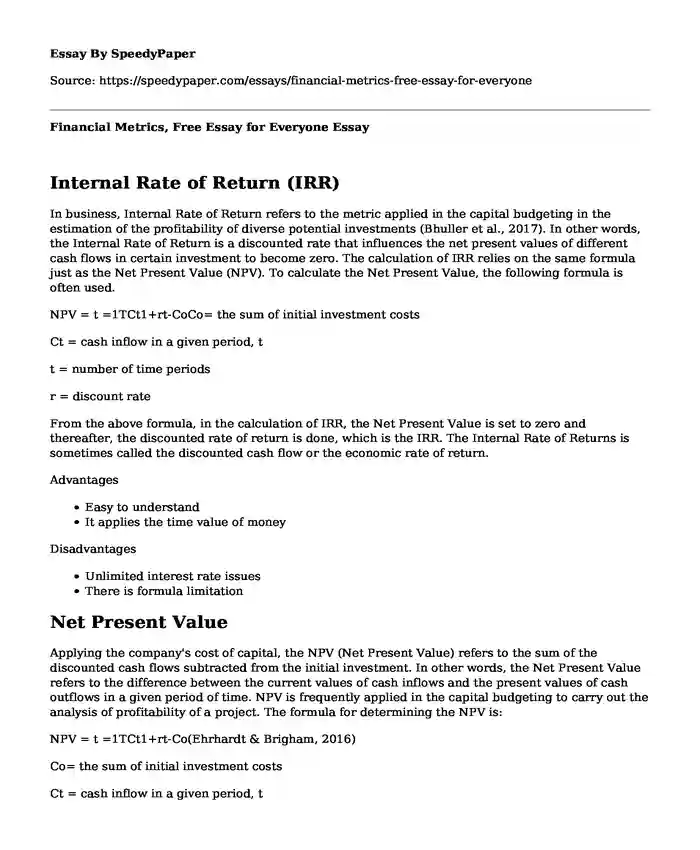# Financial Metrics, Free Essay for Everyone

Published: 2022-07-11Type of paper: Essay Categories: Budgeting Financial analysis Pages: 3 Wordcount: 634 words
143 views

## Internal Rate of Return (IRR)

In business, Internal Rate of Return refers to the metric applied in the capital budgeting in the estimation of the profitability of diverse potential investments (Bhuller et al., 2017). In other words, the Internal Rate of Return is a discounted rate that influences the net present values of different cash flows in certain investment to become zero. The calculation of IRR relies on the same formula just as the Net Present Value (NPV). To calculate the Net Present Value, the following formula is often used.

Is your time best spent reading someone else’s essay? Get a 100% original essay FROM A CERTIFIED WRITER!

NPV = t =1TCt1+rt-CoCo= the sum of initial investment costs

Ct = cash inflow in a given period, t

t = number of time periods

r = discount rate

From the above formula, in the calculation of IRR, the Net Present Value is set to zero and thereafter, the discounted rate of return is done, which is the IRR. The Internal Rate of Returns is sometimes called the discounted cash flow or the economic rate of return.

• Easy to understand
• It applies the time value of money

• Unlimited interest rate issues
• There is formula limitation

## Net Present Value

Applying the company's cost of capital, the NPV (Net Present Value) refers to the sum of the discounted cash flows subtracted from the initial investment. In other words, the Net Present Value refers to the difference between the current values of cash inflows and the present values of cash outflows in a given period of time. NPV is frequently applied in the capital budgeting to carry out the analysis of profitability of a project. The formula for determining the NPV is:

NPV = t =1TCt1+rt-Co(Ehrhardt & Brigham, 2016)

Co= the sum of initial investment costs

Ct = cash inflow in a given period, t

t = number of time periods

r = discount rate

• The cash flow from the future time is often discounted back to the present to determine the net worth
• Stockholders can clearly see the value of their investments
• The computation applies the cost of capital as the discounted rate

• It requires guessing about the future
• It is not applicable when comparing projects with different investments
• The NPV approach is not viable while comparing diverse projects

## Payback Method

The payback period refers to the duration of time needed to recover the costs of investments. The payback period of a project or a given investment is an essential determinant of whether to engage in taking the position or the actual investment (Sobel et al., 2009). In many cases, longer payback periods are not desirable for investment positions.

• Useful for risk analysis
• Gives a quick picture for the time that the initial investment will be at risk
• It is useful in organizations where the investments become outdated so quickly

• Cash flow complexities
• The method does not take into account the time value of money
• It leads to incorrect averaging

Calculations

A=p(1+rn)nt500,000 = P (1 +0.15)201

500,000 =16.37p

Therefore, p = 500,000/16.37 = 30,543.70

There is the need to deposit \$ 30, 543.70 today

ii. Future value is given by:

A=p(1+rn)nt = 200,000 (1+0.05/1) 51

= \$25, 5256.31

The future value is \$25, 5256.31

iii. Interest rate

300,000 = 100,000 (1 + r/1)10

300,000 =100,000 (1+r)10

Log(3) = log(1+r)10

0.477 = 1 +r

R =0.523

Therefore r = 52.3%

iv. A = p(1+r/n)nt

= 50,000 (1+0.11)10

= \$ (141,971.05-50,000)

= \$91,971.05

v.) 400,000 = 10,000(1+r/n)nt

log40 = log (1+r)20

Rate is 16 %

Project Cash Flows

Project ANPV = t =1TCt1+rt-Co 5000(3) = \$15,000

NPV = 15,000 -10,000 = 5,000

Project B

NPV = t =1TCt1+rt-Co = 20,000(3) - 55,000

= 60,000 - 55,000

NPV= 5,000

From the above analysis, I would select Project A because the payback period is shorter.

References

Bhuller, M., Mogstad, M., & Salvanes, K. G. (2017). Life-cycle earnings, education premiums, and internal rates of return. Journal of Labor Economics, 35(4), 993-1030.

Ehrhardt, M. C., & Brigham, E. F. (2016). Corporate finance: A focused approach. Cengage learning.

Sobel, M. J., Szmerekovsky, J. G., & Tilson, V. (2009). Scheduling projects with stochastic activity duration to maximize expected net present value. European Journal of Operational Research, 198(3), 697-705.

Financial Metrics, Free Essay for Everyone. (2022, Jul 11). Retrieved from https://speedypaper.com/essays/financial-metrics-free-essay-for-everyone

Request Removal

If you are the original author of this essay and no longer wish to have it published on the SpeedyPaper website, please click below to request its removal:

Liked this essay sample but need an original one?

Hire a professional with VAST experience!